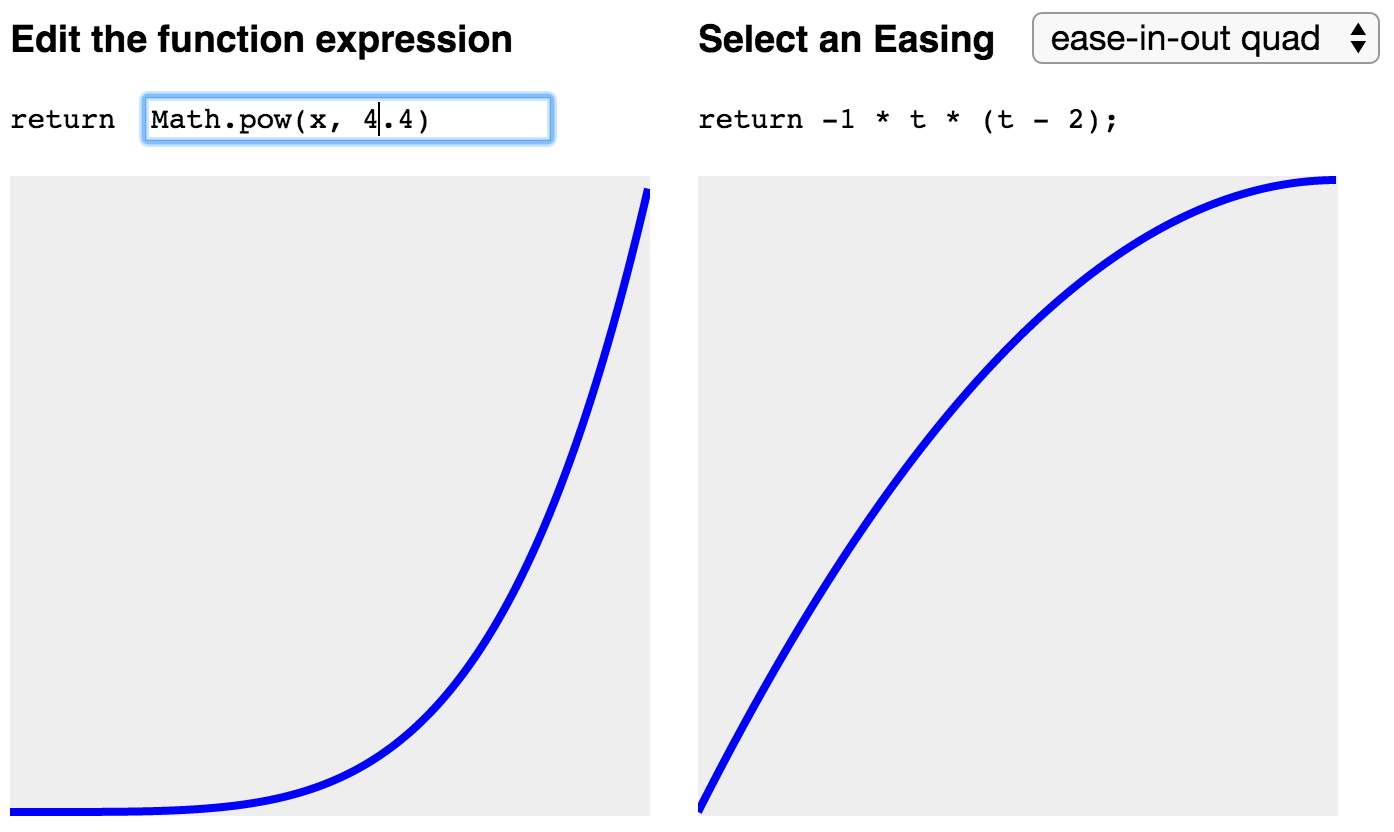# react-function-plot

react component to plot a function as an svg path

## Usage no npm install needed!

``````<script type="module">
import reactFunctionPlot from 'https://cdn.skypack.dev/react-function-plot';
</script>``````

## react-function-plot

A React component to render a JS function as an SVG path

### Install

``````npm install react-function-plot
``````

### Usage

``````// commonJS...
var Plot = require('react-function-plot');
// ...or es2015
import Plot from 'react-function-plot';

<Plot
className='myPlot'
fn={myFunction}
thickness={4}
/>
``````

The SVG element will autosize (and auto-center) within the Plot component. (The SVG container must be a square, so the height and width of the SVG element will be set to the lesser of the height and width of the Plot component)

`fn` can be any JavaScript function that takes a single numeric argument...

``````// es5
function(t) {return 0.9/t}
// es2015
x => x * x
// or even
n => Math.random();
``````

`className` is a required prop – it allows multiple `Plot` components to be used on the same page.

### Usage Example

Click here to play with a working example. Here's the source for the example component.### Tests

``````npm test
``````

(test coverage very limited so far)